# Discussion of article "A scientific approach to the development of trading algorithms"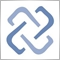Moderator
228713

New article A scientific approach to the development of trading algorithms has been published:

The article considers the methodology for developing trading algorithms, in which a consistent scientific approach is used to analyze possible price patterns and to build trading algorithms based on these patterns. Development ideals are demonstrated using examples.

Testing was performed in the period from 01.01.2018 to 28.07.2020, on the M1 timeframe, using the real tick mode. Parameters were not optimized, because I want to show that there can be no need to optimize a thoroughly prepared algorithm for each individual currency pair. We will change block size, minimum block size and lot, in an effort to have profit significantly exceeding commission size.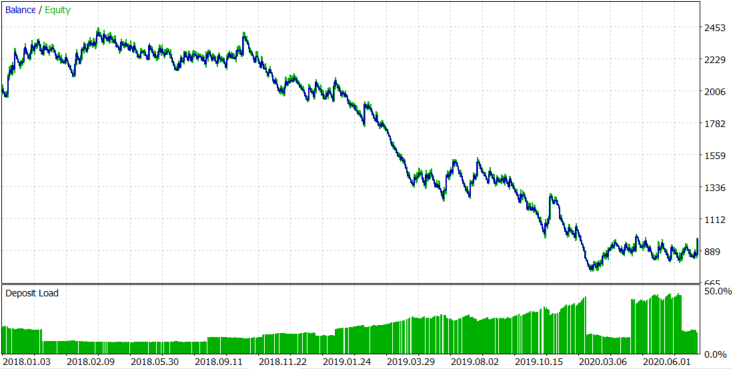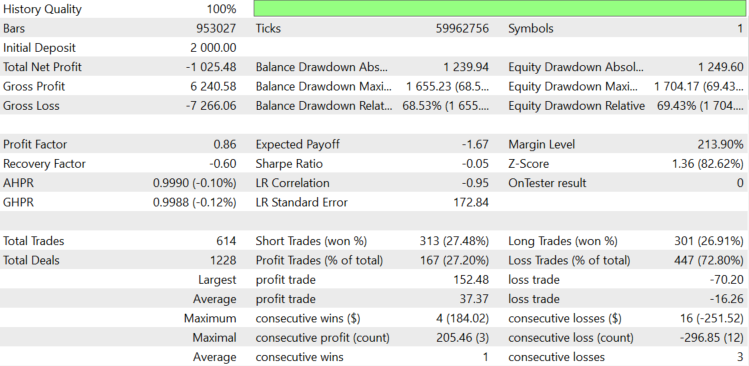Figure 7.

For EURUSD, as expected, spread and delta took all the profit that we should have received from the asset trend. As a result, the expected payoff us -\$1.67 per trade. The lot was changed dynamically, depending on the block size, with the average lot being 0.078. Let us try to understand where the loss comes from. The robot logs information about the spread. The average spread during position opening and closing is 0.00008. We paid swaps of \$159.76, and opened 614 positions. So, the average swap per position was 159.76/614=\$0.2602.

If the average spread is 0.00008 and the average lot is 0.078, 1 EURUSD pip with a lot of 0.078 is equal to \$0.078, and so spread costs 0.078*8=\$0.624. In total, the commission is equal to \$0.624+\$0.2602=\$1.104. If we were losing a commission on each deal, the expected payoff would be -\$1.104, but it is \$1.67, which is \$0.566 more. The minimum block size is set to 0.002 in settings, so it makes \$15.6 for an average lot of 0.078. Let us roughly estimate the negative balance change if the balance chart were a random walk and the block size was always minimal. It is calculated as 15,6*(614^0.5)=386.55\$. Now, add the average commission per trade multiplied by the number of trades. 1.104*614+386.55=\$1064.406.

The value is equal to \$1064.406, which means the average drawdown of a balance chart if the probability of position opening in the right direction is 50% and a commission is paid for each open position. In reality, we received a loss of \$1027.45, which is close to this value. We can conclude that we had a loss, because the expected payoff of our algorithm is zero for EURUSD.

Let us see the results on the more trending AAPL stocks. The result is shown in Figure 8 below.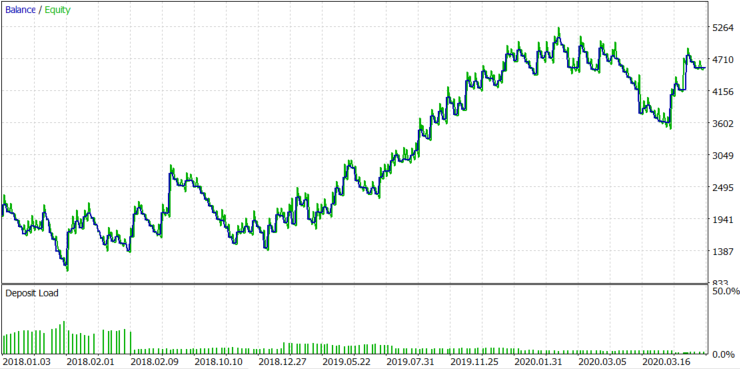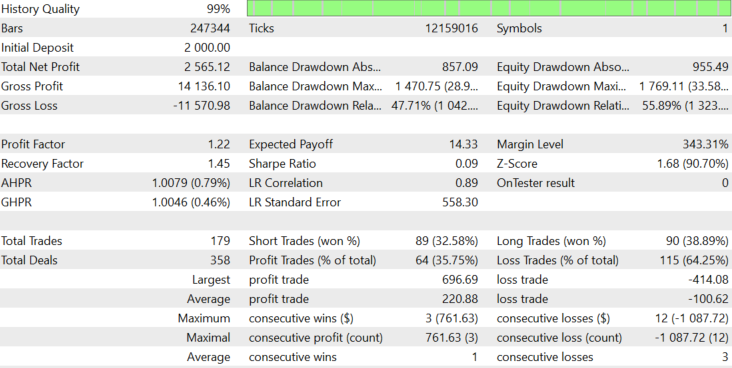Figure 8.

Author: Maxim Romanov18

MetaQuotes:

New article A scientific approach to the development of trading algorithms has been published:

Author: Maxim Romanov

Thanks for the interesting article. There is an custom indicator missing: Max_distribution_v.1.13.mq5 Can you please upload it? Thanks.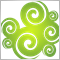9443

konorti:
Thanks for the interesting article. There is an custom indicator missing: Max_distribution_v.1.13.mq5 Can you please upload it? Thanks.

Thank you for appreciating the article. Max Distribution V.1.13.mq5 indicator I did not attach it to the article, because it is a unique, author's development and I am not ready to exhibit it for general use. <Deleted>  I warn you right away, for the robot laid out in the article, it is of no value.